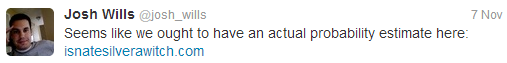# 88% chance

## Nate Silver is a witch

As Josh Wills pointed out, isnatesilverawitch.com has a dissapointing methodology: it doesn't use any statistics!• Nate's final predictions represent the true probability of Obama winning the state
• For example, Obama had a 50.3% chance of winning Florida
• Election outcomes in one state don't depend on another state
• So Obama winning Ohio doesn't effect the Nevada outcome
• If Nate Silver is a witch, then he would get every state correct
• In Bayesian terms, $$P(\text{AllStatesRight} | \text{NateIsAWitch}) = 1$$
This enables us to calculate the probability of Nate Silver correctly calling all 50 states plus Washington DC. To do this, we take the product of the probababilities the model assigned to the eventual winner of each state.

This probability was calculated by executing the following javascript code on fivethirtyeight.com:

nytg.stateData.forecast.states
.map(function(x) {return {"stateAbbreviation": x, "pObamaWinsState": x/100};})
.filter(function(x) {return isNaN(parseInt(x.stateAbbreviation));})
.map(function(x) {return [x.pObamaWinsState, 1-x.pObamaWinsState].max();})
.reduce(function(x,y) {return x*y;})


This tells us Nate Silver had a 12.53% chance of correctly calling each state.

From there, we have a straightforward application of Bayes theorem: $$P(H|D)=\frac{P(D|H)P(H)}{P(D)}=\frac{P(D|H)P(H)}{P(D|H)P(H)+P(D|H')P(H')}$$ with \begin{align*}&H \text{, the hypothesis that Nate Silver is a witch}~\\ &D \text{, the data that Nate accurately predicted all states}~\\ &P(D|H) = 1 \text{ (if Nate was a witch he would've nailed each state)}~\\ &P(D|H') = 0.1253 \text{ (probability of Nate predicting all states given our model)}~\\ &P(H) \text{, your prior belief that Nate is a witch}~\\ &P(H') = 1-P(H)\end{align*} Thus, we come to a different conclusion - Nate Silver is probably not a witch for any reasonable priors.